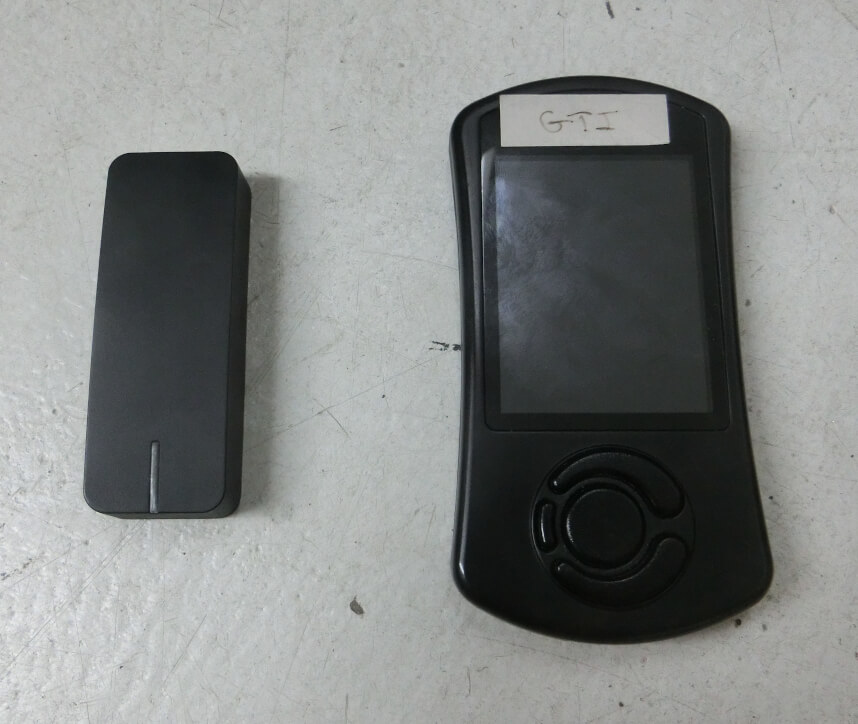# Dragy vs Cobb AP

## Background:

Normally I record vehicle performance data using the Cobb AP. One value that is recorded is engine RPM and I will calculate the time for the GTI to accelerate from 3500 RPM to 6300 RPM to be used for comparing the results of changes, such as tune revisions or swapping parts.

Also available is a Dragy data logger and it can be configured to record custom time intervals between designated speeds.

3500 RPM equates to 47 MPH, with my 6-speed DSG equipped GTI, and 6300 RPM equates to 84 MPH.

This is an evaluation to see how closely the two recording devices are at measuring acceleration times.

## Test Procedures:

The Cobb AP is attached to the OBD port and logs the engine speed with a timestamp while the Dragy is set to record the time between 47-84 MPH.

Beginning below 47 mph the GTI is accelerated at full throttle in third gear several times across the speed range concluding just past 84 mph.

The data from the Cobb AP is used to calculate the time to accelerate. Because the AP samples are at approximately 40 Hz the starting and ending engine speeds are sometimes not recorded exactly at 3500 and 6300 RPM and a linear interpolation is performed to find a starting and ending time. For example:

With the resulting times:

The difference in start and end times is calculated.

Note: If the values were expressed out to three decimal places they would show a start at 439.782 and finish at 443.587.

The Dragy samples at 10 Hz and performs an internal interpolation so that it reports a single time for the acceleration between the designated speeds.

The GTI is accelerated 8 times.

## Test Results:

Data recorded by each device is shown in the table:

Comparing the data with a box plot:

Interestingly the Cobb data shows less variation than the Dragy. Possibly a result of the Cobb having a higher sampling rate.

Evaluating the data with a t-test. The calculated variance confirms what is shown by the box plot, the Cobb has “much less” variance across the data samples.

Much less being relative, the variance by the Dragy is just 1/100th of a second.

The results of the t-test, which is hypothesizing that the average values from each device are the same, cannot be rejected.

This data does not show a significant difference in the average acceleration time reported by either device.

## Conclusion:

The Dragy and Cobb AP measured equivalent acceleration times for the GTI . Either device can be used to record acceleration times for the purpose of comparing acceleration performance from 47 to 84 mph as described in this post.